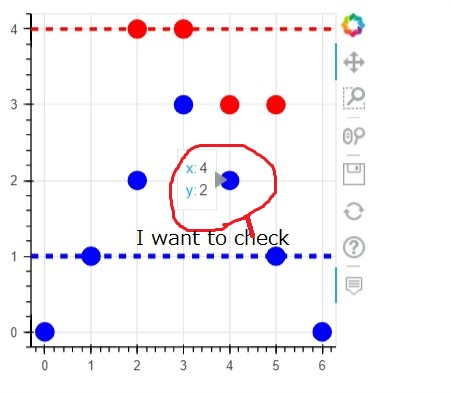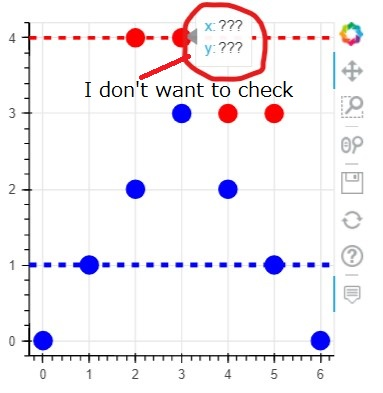How can I hide hover value in dual plots

Hi all,

I am making two plots in one figure. And I use hover tool. I want to check value in one plot(blue points). and don’t want to check value in other plot(red points) using hover tool like as below.

output screen 1 [I want to check blue points value]output screen 2 [I don’t want to check red points value]My simple code is below.

from bokeh.models import HoverTool,Span
from bokeh.plotting import ColumnDataSource, figure , show
import pandas as pd; import numpy as np

x1 = [0, 1, 2, 3, 4, 5, 6]
y1 = [0, 1, 2, 3, 2, 1, 0] # <-- I want to check
x2 = [2, 3, 4, 5]
y2 = [4, 4, 3, 3]  # <-- I don't want to check

temp_data = pd.DataFrame(data=[x1,y1])
disp_data = temp_data.T
disp_data.columns =['X1','Y1']
t_src = ColumnDataSource(disp_data)

# I want to check blue data using hover tool
p1 = figure(width=300, height=300, name='Scatter')
p1.scatter(x='X1',y='Y1' , color = 'blue' , source=t_src , size = 15)
# I don't want to check red  data using hover tool
p1.scatter(x2,y2 , color = 'red' , size = 15)

hline = Span(location=4 , dimension='width', line_color='red', line_width=3 , line_dash='dashed')
p1.renderers.extend([hline]) #add line to the plot (Hi-Limit)

lline = Span(location=1 , dimension='width', line_color='blue', line_width=4 , line_dash='dashed')
p1.renderers.extend([lline]) #add line to the plot (Lo-Limit)

hover = HoverTool(tooltips=[('x', '@X1'), ('y', '@Y1')], mode='mouse')

show(p1)

Could you tell me how can I hide hover value on red points in this graph? I want to check blue points only.

@kazux68k

The HoverTool has a renderers argument to control which glyphs include hover information.

Here’s your code with a renderer for the blue-circle scatter points and the HoverTool configured to use only it.

#!/usr/bin/env python3
# -*- coding: utf-8 -*-
"""
"""

from bokeh.models import HoverTool,Span
from bokeh.plotting import ColumnDataSource, figure , show
import pandas as pd; import numpy as np

x1 = [0, 1, 2, 3, 4, 5, 6]
y1 = [0, 1, 2, 3, 2, 1, 0] # <-- I want to check
x2 = [2, 3, 4, 5]
y2 = [4, 4, 3, 3]  # <-- I don't want to check

temp_data = pd.DataFrame(data=[x1,y1])
disp_data = temp_data.T
disp_data.columns =['X1','Y1']
t_src = ColumnDataSource(disp_data)

# I want to check blue data using hover tool
p1 = figure(width=300, height=300, name='Scatter')
r1 = p1.scatter(x='X1',y='Y1' , color = 'blue' , source=t_src , size = 15)
# I don't want to check red  data using hover tool
p1.scatter(x2,y2 , color = 'red' , size = 15)

hline = Span(location=4 , dimension='width', line_color='red', line_width=3 , line_dash='dashed')
p1.renderers.extend([hline]) #add line to the plot (Hi-Limit)

lline = Span(location=1 , dimension='width', line_color='blue', line_width=4 , line_dash='dashed')
p1.renderers.extend([lline]) #add line to the plot (Lo-Limit)

hover = HoverTool(renderers=[r1], tooltips=[('x', '@X1'), ('y', '@Y1')], mode='mouse')
Thank you for your quick reply and suggest me good solution. I modified my code. And my program works what I wanted. I appreciate you.